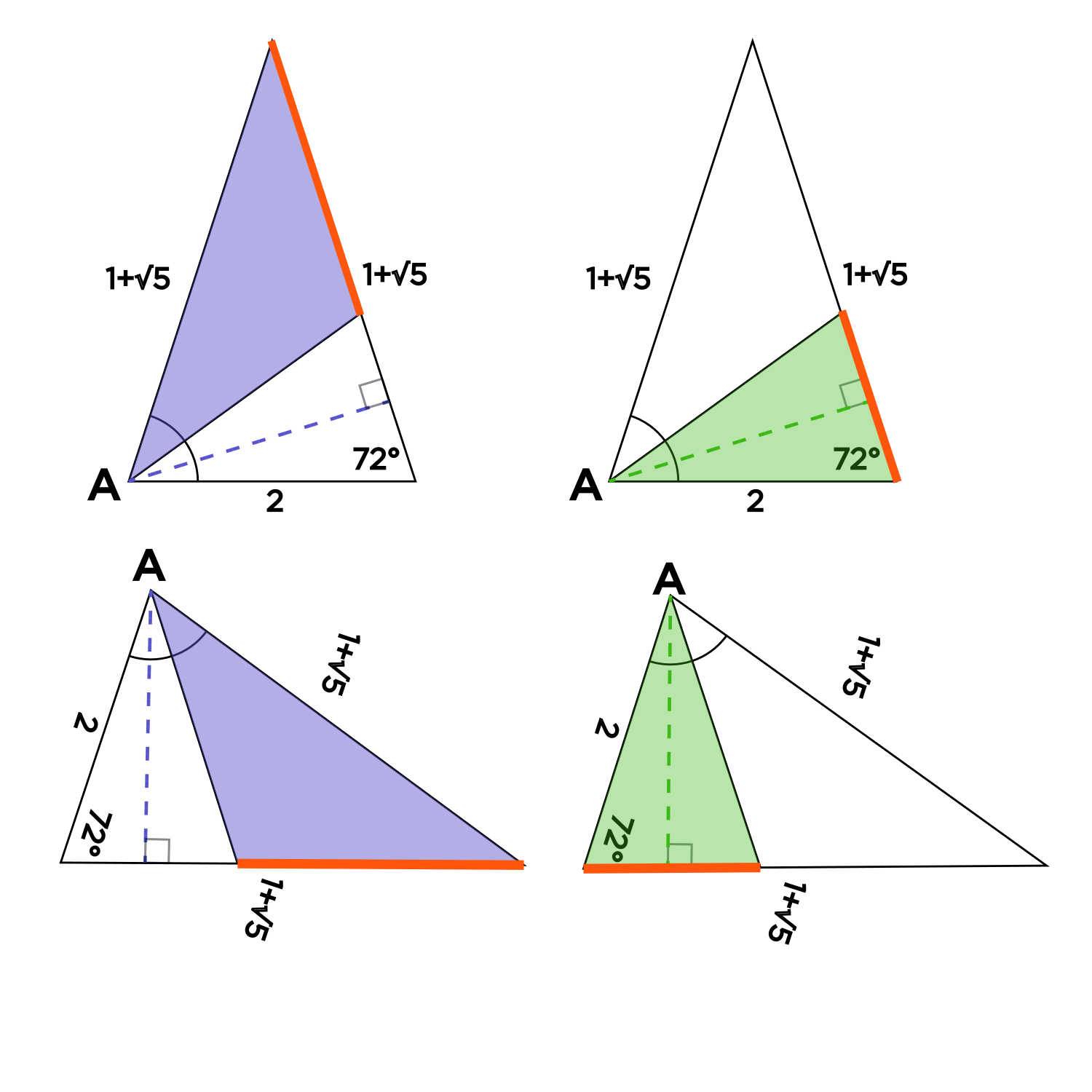# Why do the two triangles have the same height?

• This post is deleted!

• @CoherentMango Thanks for asking!This is true whenever you have two triangles that share a baseline and a common vertex that meets the altitude. I've colored the baseline $$\textcolor{red}{\text{ red }}$$ below. The common vertex is labeled $$A.$$

The obtuse $$\textcolor{purple}{\text{ purple }}$$ triangle has an altitude which is perpendicular to the baseline, emanating from point $$A,$$ shown as the dotted $$\textcolor{purple}{\text{ purple }}$$ line. Similarly, the green acute triangle has its altitude perpendicular to the baseline and emanating from the point $$A,$$ shown as a dotted $$\textcolor{green}{\text{ green } }$$ line.Turned around, it might be easier to see that they share a common baseline and altitude!